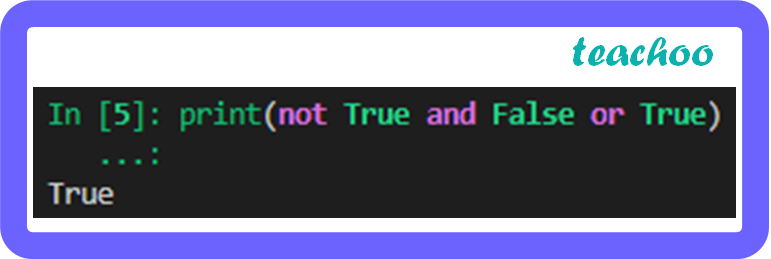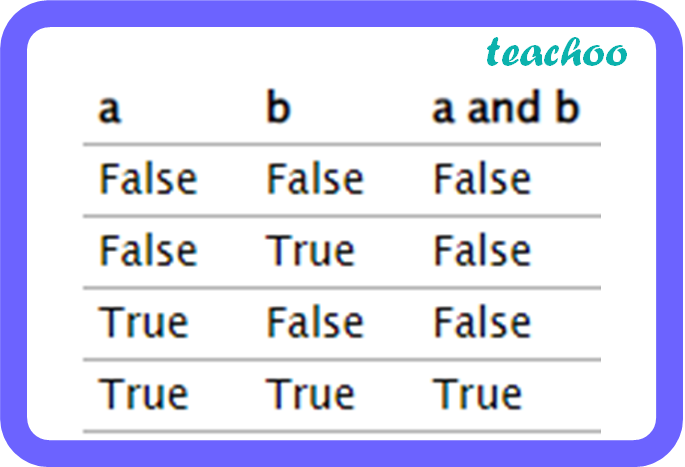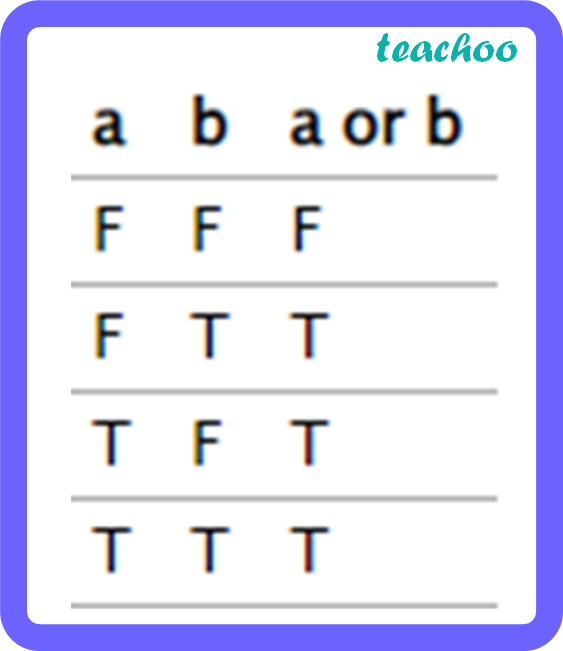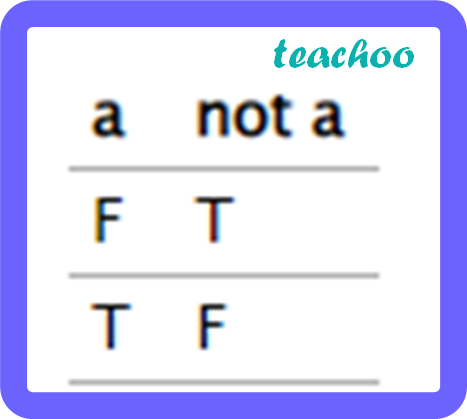CBSE Class 12 Sample Paper for 2023 Boards

Computer Science - Class 12
Solutions to CBSE Sample Paper - Computer Science Class 12

## (d) NULLOn evaluating the given expression, the output given is True .

So, the correct answer is (a).

Explanation:

And, or and not are the three logical operators in the above expression.

The truth tables for the 3 operators are:

a. andb. orc. notEvaluating the given expression:

Step 1: not True gives False. So the expression becomes ‘ False and False or True ’.

Step 2: False and False give False. So the expression becomes ‘ False or True ’.

Step 3: False or True gives True. So the expression becomes ‘ True’.

Therefore, the output will be True if the given expression is evaluated.

Learn in your speed, with individual attention - Teachoo Maths 1-on-1 Class